[an error occurred while processing this directive] [an error occurred while processing this directive]### Question of the Day

In how many ways can you draw a card from a standard deck of 52? In how many ways can you draw a pair of cards from a standard deck of 52? In how many ways can you draw three cards from a deck of 52 cards? In the last two cases, assume that order does not matter. Discuss why it is safe to assume that order does not matter.### Key Concepts

1. The Generalized Principle of Counting says that if there are m ways for a first action to occur and then there n ways for a second action to occur, then altogether there are m . n ways for the pair of actions to occur (in order.)
2. Counting is hard, so it is important to look at simple cases, and apply the methods systematically to more complex cases.### Vocabulary

1.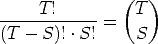is the number of combinations of S objects taken from a larger set of T objects.

2. The expression or symbol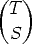is called a combination. It is also called a binomial coefficient, since it occurs in the expansion of a binomial expression.### Mathematical Ideas

#### Combinations

Consider the number of ways of selecting a subset of S things from a larger collection of T things. By the Fundamental Principle of Counting, there are T ways of selecting the first thing, then T - 1 ways of selecting the second thing, so there are T . (T - 1) ways of selecting both. Extending this idea, there are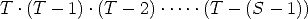ways of selecting the S objects. But this counts order. There are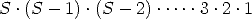ways of ordering the S objects in a row. Hence bunching or collecting the selections of S objects in groups with the same elements in all the possible orders, we see that there is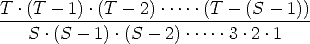ways of selecting S objects from a larger group of T objects, when the section is without regard to order.

This calculation can be represented as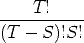Note the cancellation of the factors (T - S), (T - S - 1) . . . 3, 2, 1 from numerator and denominator to leave just the expression above.

This expression is so common, it is given a name and a symbol: We write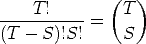and we say that this is the number of combinations of S objects taken from a larger set of T objects. The expression or symbol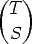is called a combination. It is also called a binomial coefficient, since it occurs in the expansion of a binomial expression.

The binomial coefficients occur in many places in mathematics. In particular, the entry in the S position across in the T row of Pascal’s Triangle is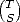.

### Sample Worked Problems

#### Problem 14, Oregon Lotto

Problem Statement: To win the jackpot of the Oregon lottery game, you must correctly pick the six numbers selected fromt he numbers 1 through 42. What is the probability of winning the Oregon Lottery?

Comment: As of March 2006, these rules do not seem to be the Oregon lottery anymore. It appears that Oregon now plays a number of games, including the closest which is the now multi-state PowerBall. For PowerBall one must match 5 numbers drawn from a set of balls numbered 1 to 55, and a sixth PowerBall drawn from a separate set of balls numbered 1 to 42.

But anyway, assuming the rules above, there are 42 ways to draw the first ball, then 41 ways to draw the second ball, and so on, down to 42 - (6 - 1) = 37 ways to draw the sixth ball. There are 42 . 41 . 40 . 39 . 38 . 37 = 3776965920 ways of drawing the 6 numbers. However, the order of drawing the balls does not matter, only the numbers themselves (which are usually displayed in increasing order for convenience in matching.) Given a set of 6 distinct numbers there would be 6! = 720 ways of ordering them. Each of these ways would be enumerated in the ordered listing, but all would be equivalent in the rules of the lottery. Hence, we must divide 3776965920/720 = 5245786 to get the number of equivalent drawings. Only one of these would match our pick of six numbers, so the chance of winning the lottery in these rules is 1/5245786, that is, less than one in 5 million!

#### Problem 33, Car Care

Problem Statement: A burglar wishes to break into a car that has a security system. There are 5 buttons marked 1 through 5 to disarm he system and he knows the code is a three-digit number. How many possible security codes are there? Knowing this number of combinations, the burglar fears that he will enter the wrong number first - thus tripping the alarm (which everyone just ignores). SO he writes down his first 20 guesses and then enters his next guess. Does this strategy improve his chances of success?

Solutions: We must make an assumption that numbers in the security code may be repeated and we may safely assume that order counts. Thus, 123 is a possible security code and 321, 231 and 132 are all distinct possible security codes. Likewise, 555 is also a possible security code. There are 5 choices for the first code number, then 5 choices for the second code number for a possible total of 5 . 5 choices for the first two numbers. Then there are 5 more choices for the third code number for a total of 5 . 5 . 5 = 125 possible codes.

The burglar’s strategy does not pay off in increased chances of disarming the system. Using the 21st guess after abandoning the first twenty guesses is no better than using the first guess. Another way of saying it is the guesses are independent, and so each new guess starts the process over, without altering the probability of success at any stage.

#### Problem 30, Making up the Test

Problem Statement: Your math prof says there will be a 15-question test on probability. She also reports that the test will be made up of problems from the Problem Sets of the Chapter as follows: two questions each from Sections 7.2, 7.3, and 7.7 (with 40, 40 and 35 questions each.) and three questions each from 7.4, 7.5, 7.6 (with 40, 22, and 35 questions each.)

Solutions: Then there will be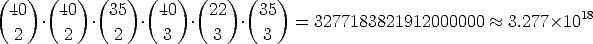possible tests.

### Problems to Work for Understanding

1. Solidifying Ideas: pages 566: 11,12,13,23,25
2. New Ideas: page 568: 31, 32
3. Habits of Mind: page 569: 36